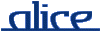# alice library manual.# The MONO_VECTOR signature

## ________ Synopsis ____________________________________________________

```    signature MONO_VECTOR
structure CharVector : MONO_VECTOR where type elem = char
structure Word8Vector : MONO_VECTOR where type elem = Word8.word
```

An extended version of the Standard ML Basis' MONO_VECTOR signature.

## ________ Import ______________________________________________________

Imported implicitly.

## ________ Interface ___________________________________________________

```    signature MONO_VECTOR =
sig
eqtype vector
type   t = vector

val maxLen :      int

val toList :      vector -> elem list
val fromList :    elem list -> vector
val tabulate :    int * (int -> elem) -> vector

val length :      vector -> int
val sub :         vector * int -> elem
val update :      vector * int * elem -> vector
val concat :      vector list -> vector
val rev :         vector -> vector

val app :         (elem -> unit) -> vector -> unit
val appr :        (elem -> unit) -> vector -> unit
val map :         (elem -> elem) -> vector -> vector
val foldl :       (elem * 'a -> 'a) -> 'a -> vector -> 'a
val foldr :       (elem * 'a -> 'a) -> 'a -> vector -> 'a
val all :         (elem -> bool) -> vector -> bool
val exists :      (elem -> bool) -> vector -> bool
val find :        (elem -> bool) -> vector -> elem option

val appi :        (int * elem -> unit) -> vector -> unit
val appri :       (int * elem -> unit) -> vector -> unit
val mapi :        (int * elem -> elem) -> vector -> vector
val foldli :      (int * elem * 'a -> 'a) -> 'a -> vector -> 'a
val foldri :      (int * elem * 'a -> 'a) -> 'a -> vector -> 'a
val alli :        (int * elem -> bool) -> vector -> bool
val existsi :     (int * elem -> bool) -> vector -> bool
val findi :       (int * elem -> bool) -> vector -> (int * elem) option

val contains :    (elem * elem -> bool) -> vector -> elem -> bool
val notContains : (elem * elem -> bool) -> vector -> elem -> bool

val equal :       (elem * elem -> bool) -> vector * vector -> bool
val collate :     (elem * elem -> order) -> vector * vector -> order

val isSorted :    (elem * elem -> order) -> vector -> bool
val sort :        (elem * elem -> order) -> vector -> vector
end
```

## ________ Description _________________________________________________

Items not described here are as in the Standard ML Basis' MONO_VECTOR signature.

Note that, unlike polymorphic vectors, monomorphic vectors are strict in their elements. I.e., all constructor functions taking individual element values (fromList, tabulate, update) will request any potential futures immediately.

type t = vector

A local synonym for type vector.

toList vec

Creates a list of the elements of vec in order of increasing indices.

rev vec

Returns a vector that contains the elements of vec in reverse order.

appr f vec
appri f vec

Like app and appi, but apply f in right to left order (i.e., decreasing indices). The expression app f vec is equivalent to:

`        appri (f o #2) vec`
alli f vec
existsi f vec

Indexed versions of the functions all and exists. The index of each element is passed to f as an additional argument. The following equivalences hold:

```        all f vec    = alli (f o #2) vec
exists f vec = existsi (f o #2) vec```
contains eq vec a

Returns true if the element a occurs in the vector vec, with respect to the element equality eq; otherwise false.

notContains eq vec a

Returns true if the element a does not occur in the vector vec, with respect to the element equality eq; otherwise false. Equivalent to not(contains eq vec a).

equal eq (vec1, vec2)

Creates an equality function on vectors given an equality on the element type.

isSorted f vec

Returns true iff vec is sorted with respect to the ordering function f.

sort f vec

Returns a new vector that contains the same elements as vec, but sorted with respect to the ordering function f. Sorting may be unstable with respect to equal elements.